Courses

# Test: Distance Formula

## 20 Questions MCQ Test Mathematics (Maths) Class 10 | Test: Distance Formula

Description
This mock test of Test: Distance Formula for Class 10 helps you for every Class 10 entrance exam. This contains 20 Multiple Choice Questions for Class 10 Test: Distance Formula (mcq) to study with solutions a complete question bank. The solved questions answers in this Test: Distance Formula quiz give you a good mix of easy questions and tough questions. Class 10 students definitely take this Test: Distance Formula exercise for a better result in the exam. You can find other Test: Distance Formula extra questions, long questions & short questions for Class 10 on EduRev as well by searching above.
QUESTION: 1

Solution:
QUESTION: 2

### One end of a line of length 10 units is at the point (-3, 2). If the ordinate of the other end be 10, then the abscissa will be

Solution: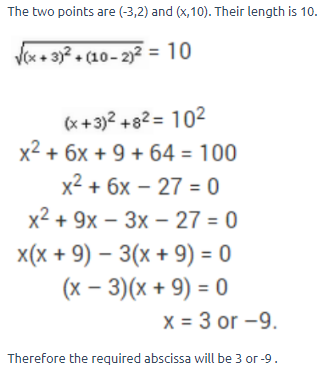QUESTION: 3

### The horizontal and vertical lines drawn to determine the position of a point in a Cartesian plane are called​

Solution:

The point is determined on 2 dimensions .The name of horizontal and vertical lines drawn to determine the position of any point in the Cartesian plane is x-axis and y-axis respectively. The name of each part of the plane formed by these two lines x-axis and the y-axis is quadrants. The point where these two lines intersect is called the origin.

QUESTION: 4

Let P(x, y) be equidistant from the points A (7, 1) and (3, 5).Find a relation between x and y.​

Solution:
QUESTION: 5

If the four points (0,-1), (6,7),(-2,3) and (8,3) are the vertices of a rectangle, then its area is​

Solution:

Let A(0-1), B(6,7), C(-2,3) and D(8,3) be the given points. Then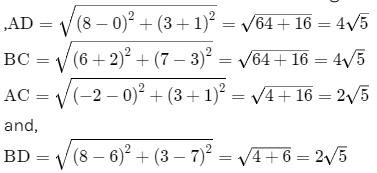∴ AD = BC and AC = BD

Now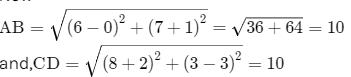Clearly, AB= AD+ DBand CD= CB+ BD2

Now

,Area of rectangle ADBC = AD × DB =(4√5​ × 2√5​)sq. units = 40sq. units

QUESTION: 6

The perimeter of a triangle with vertices (0, 4) (0, 0) and (3, 0) is:

Solution:
QUESTION: 7

The ordinate of a point is twice its abscissa. If its distance from the point (4,3) is √10,  then the coordinates of the point are​

Solution:
QUESTION: 8

The perimeter of the triangle formed by the points A(0,0), B(1,0) and C(0,1) is

Solution:
QUESTION: 9

The value of k, if the point P(0,2) is equidistant from A(3,k) and B(k,5) is

Solution:
QUESTION: 10

If A and B are the points (-6, 7) and (-1, -5) respectively, then the distance 2AB is equal to​

Solution:
QUESTION: 11

The values of x and y, if the distance of the point (x,y) from (-3,0) as well as from (3,0) is 4 are

Solution:
QUESTION: 12

The point (-1,-5) lies in the Quadrant​

Solution:
QUESTION: 13

The distance between the points (3,4) and (8,-6) is​

Solution:

Distance formula=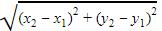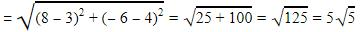QUESTION: 14

The point on y-axis that is equidistant from (2,3) and (-4,1) is​

Solution:

A(2, 3) and B(-4, 1) are the given points.

Let C(0.y) be the points are y - axis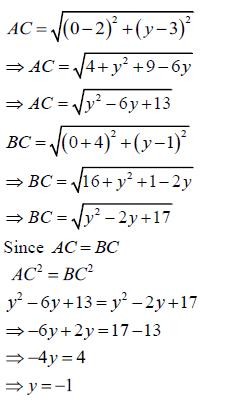Therefore, The point on y - axis is (0,-1)

QUESTION: 15

The point on x-axis which is equidistant from (5,9) and (-4,6) is​

Solution:
QUESTION: 16

The distance of the point P(6,-6) from the origin is equal to​

Solution:
QUESTION: 17

The distance between the points P (-6,7) and Q (-1,-5) is​

Solution:
QUESTION: 18

The condition that the point (x,y) may lie on the line joining (3,4) and (-5,-6) is​

Solution:

Since the point P(x,y) lies on the line joining A(3,4) and B(-5,-6),

Therefore, points P, A and B are collinear points.

So, area of triangle PAB = 0

Therefore, we have:

10x-18-3y-5y+20=0

10x-8y+2=0

5x-4y+1=0 , which is the required condition.

QUESTION: 19

The points (3, 2), (0, 5), (-3, 2) and (0, -1) are the vertices of a quadrilateral. Which quadrilateral is it?​

Solution:
QUESTION: 20

The graph of the equation x = 3 is:​

Solution:

x=3 is fixed. This means the value of x is constant. So y can vary but x has only one value. For example (3,0),(3,2),(3,5) etc. So the line drawn will be parallel to y axis as y can vary.